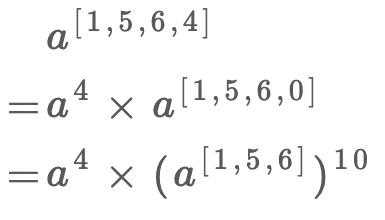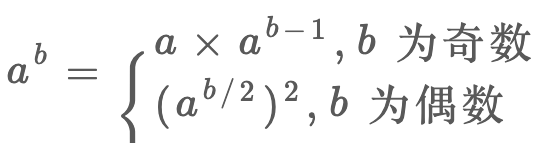• 累计撰写 270 篇文章
• 累计创建 6 个标签
• 累计收到 1 条评论

### 目 录CONTENT# 高效进行模幂运算.md2021-08-22 / 0 评论 / 0 点赞 / 9 阅读 / 2,363 字 / 正在检测是否收录...

@[toc]

## 一、题目描述

输入: a = 2, b = 



输入: a = 2, b = [1,0]



## 二、分析

•  一是如何处理用数组表示的指数，现在 b 是一个数组，也就是说 b可以非常大，没办法直接转成整型，否则可能溢出。你怎么把这个数组作为指数，进行运算呢？
•  二是如何得到求模之后的结果？按道理，起码应该先把幂运算结果算出来，然后做 % 1337 这个运算。但问题是，指数运算你懂得，真实结果肯定会大得吓人，也就是说，算出来真实结果也没办法表示，早都溢出报错了。
•  三是如何高效进行幂运算，进行幂运算也是有算法技巧的，如果你不了解这个算法，后文会讲解。

如何处理数组指数：

•  首先明确问题：现在 b 是一个数组，不能表示成整型，而且数组的特点是随机访问，删除最后一个元素比较高效
•  不考虑求模的要求，以 b = [1,5,6,4] 来举例，结合指数运算的法则，我们可以发现这样的一个规律：superPow(a, [1,5,6,4])
=>  superPow(a, [1,5,6])


// 计算 a 的 k 次方的结果
// 后文我们会手动实现
int mypow(int a, int k);

int superPow(int a, vector<int>& b)
{
// 递归的 base case
if (b.empty())
return 1;

// 取出最后一个数
int last = b.back();
b.pop_back();

// 将原问题化简，缩小规模递归求解
int part1 = mypow(a, last);
int part2 = mypow(superPow(a, b), 10);

// 合并出结果
return part1 * part2;
}


如何处理 mod 运算：

•  首先明确问题：由于计算机的编码方式，形如 (a * b) % base这样的运算，乘法的结果可能导致溢出，我们希望找到一种技巧，能够化简这种表达式，避免溢出同时得到结果。
•  比如在二分查找中，我们求中点索引时用 (l+r)/2 转化成 l+(r-l)/2，避免溢出的同时得到正确的结果。
•  那么，说一个关于模运算的技巧吧，毕竟模运算在算法中比较常见：
(a * b) % k = (a % k)(b % k) % k
证明很简单，假设：
a = Ak +B；b = Ck + D

ab = ACk^2 + ADk + BCk +BD

ab % k = BD % k

a % k = B；b % k = D

(a % k)(b % k) % k = BD % k


•  换句话说，对乘法的结果求模，等价于先对每个因子都求模，然后对因子相乘的结果再求模。
•  那么扩展到这道题，求一个数的幂不就是对这个数连乘么？所以说只要简单扩展刚才的思路，即可给幂运算求模：

## 三、代码

int base = 1337;

// 计算 a 的 k 次方然后与 base 求模的结果
int mypow(int a, int k)
{
// 对因子求模
a %= base;
int res = 1;
for (int _ = 0; _ < k; _++)
{
// 这里有乘法，是潜在的溢出点
res *= a;

// 对乘法结果求模
res %= base;
}
return res;
}

int superPow(int a, vector<int>& b)
{
if (b.empty())
return 1;

int last = b.back();
b.pop_back();

int part1 = mypow(a, last);
int part2 = mypow(superPow(a, b), 10);

// 每次乘法都要求模
return (part1 * part2) % base;
}

•  你看，先对因子 a 求模，然后每次都对乘法结果 res 求模，这样可以保证 res *= a 这句代码执行时两个因子都是小于base 的，也就一定不会造成溢出，同时结果也是正确的。
•  至此，这个问题就已经完全解决了，已经可以通过 LeetCode 的判题系统了。
但是有的读者可能会问，这个求幂的算法就这么简单吗，直接一个 for 循环累乘就行了？复杂度会不会比较高，有没有更高效地算法呢？
•  有更高效地算法的，但是单就这道题来说，已经足够了。
•  因为你想想，调用 mypow 函数传入的 k 最多有多大？k 不过是 b 数组中的一个数，也就是在 0 到 9 之间，所以可以说这里每次调用 mypow 的时间复杂度就是 O(1)。整个算法的时间复杂度是 O(N)，N 为 b 的长度

如何高效求幂：•  这个思想肯定比直接用 for 循环求幂要高效，因为有机会直接把问题规模（b 的大小）直接减小一半，该算法的复杂度肯定是 log级了。
•  那么就可以修改之前的 mypow 函数，翻译这个递归公式，再加上求模的运算：
int base = 1337;

int mypow(int a, int k)
{
if (k == 0)
return 1;

a %= base;

if (k % 2 == 1)
{
// k 是奇数
return (a * mypow(a, k - 1)) % base;
}
else
{
// k 是偶数
int sub = mypow(a, k / 2);
return (sub * sub) % base;
}
}

0